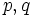# Group whose order has at most two prime factors

This article defines a property that can be evaluated for finite groups (and hence, a particular kind of group property)
View other properties of finite groups OR View all group properties

## Definition

A group whose order has at most two prime factors is a finite group such that there are at most two prime numbers which divide the order of the group. In other words, the order of the group has the form$p^aq^b$ where$p,q$ are prime numbers and$a,b$ are nonnegative integers.